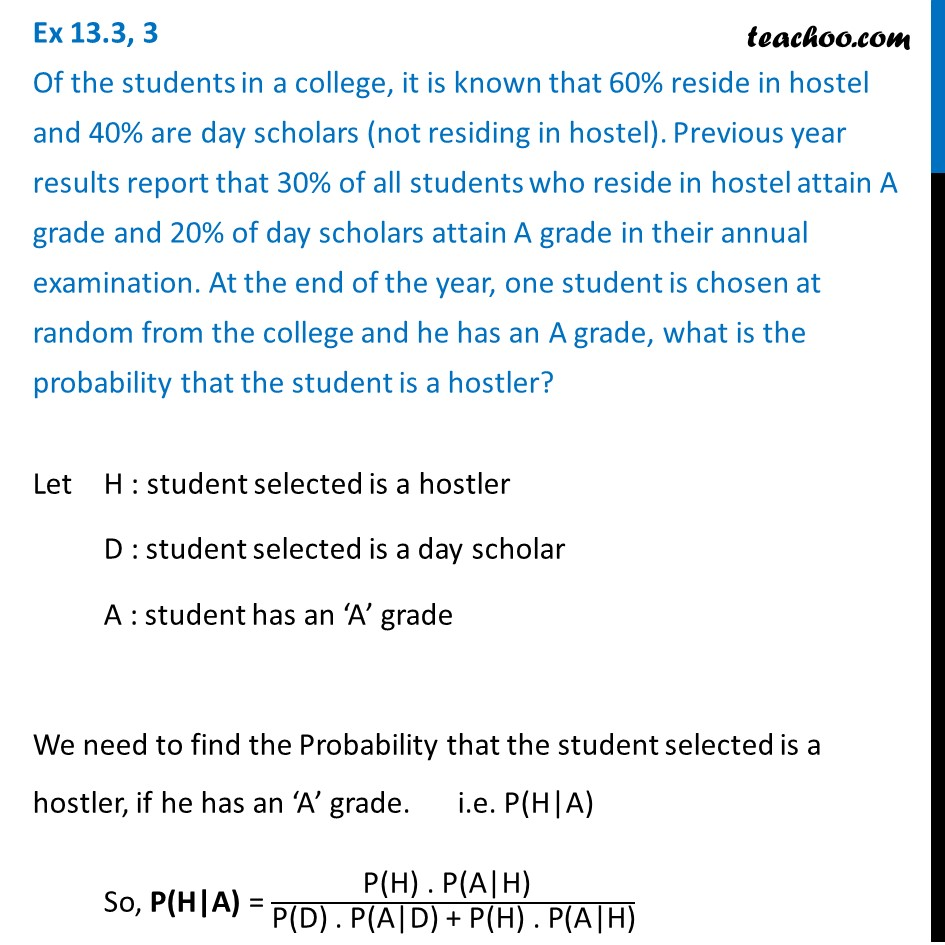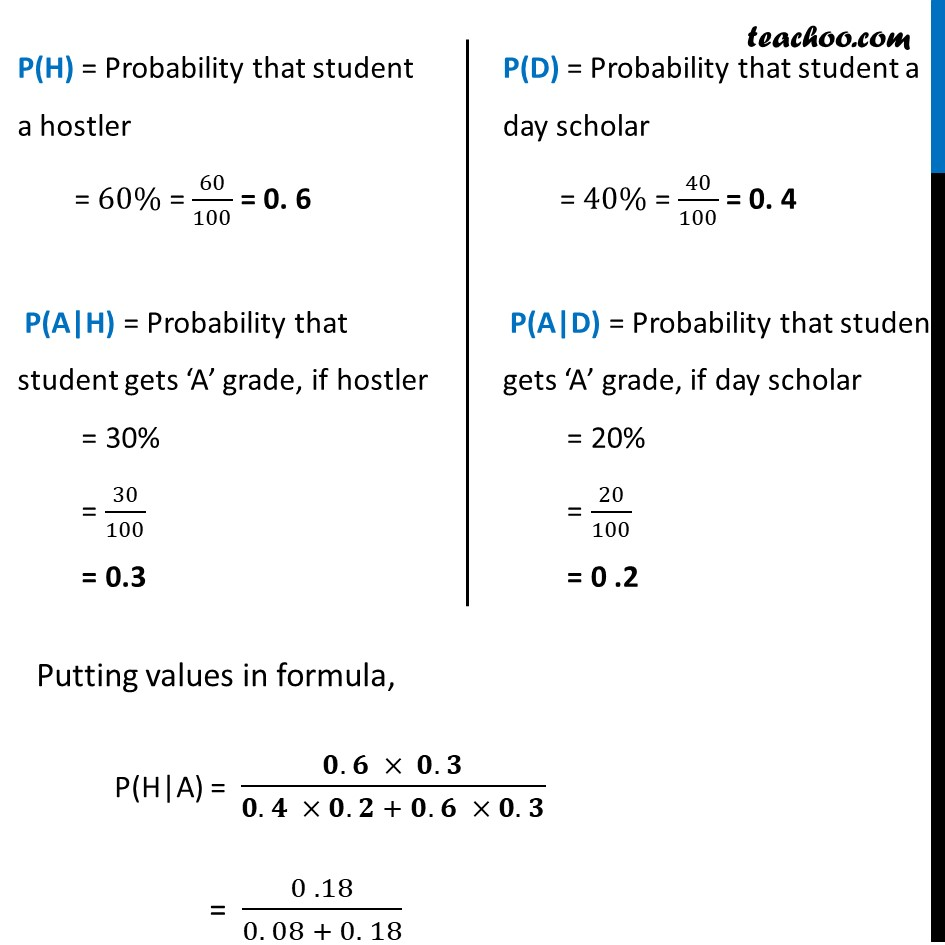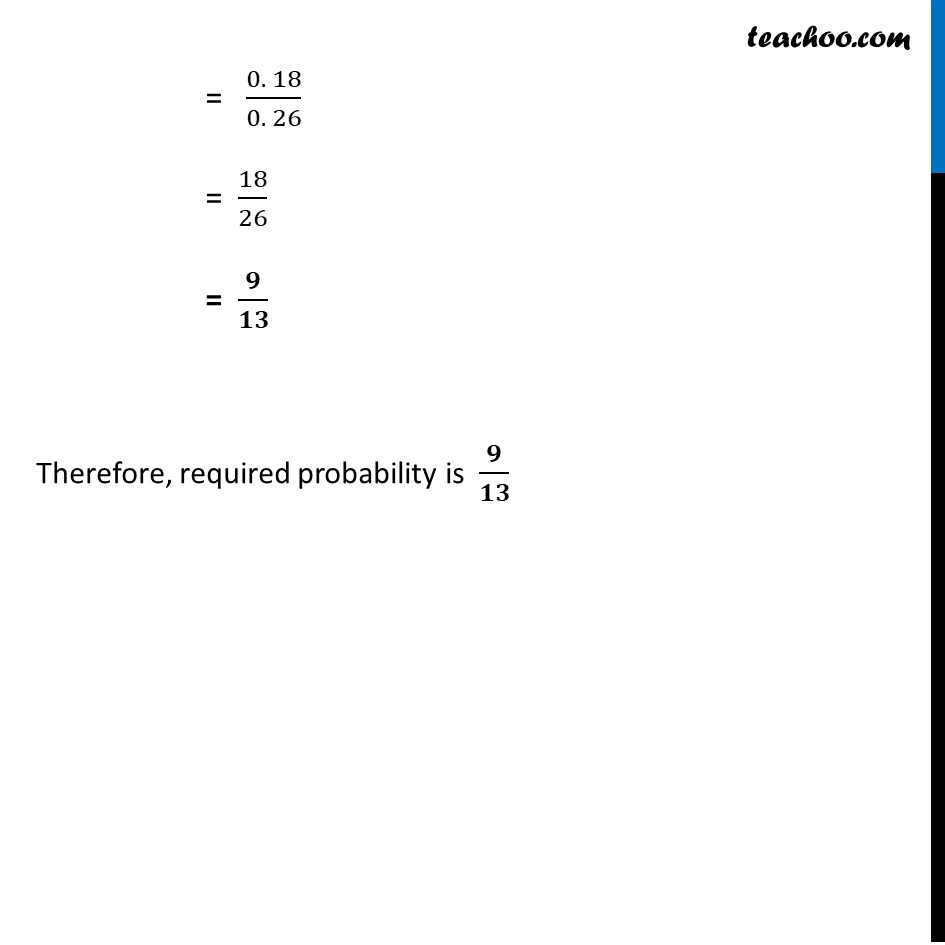Ex 13.3

Chapter 13 Class 12 Probability
Serial order wiseLearn in your speed, with individual attention - Teachoo Maths 1-on-1 Class

### Transcript

Ex 13.3, 3 Of the students in a college, it is known that 60% reside in hostel and 40% are day scholars (not residing in hostel). Previous year results report that 30% of all students who reside in hostel attain A grade and 20% of day scholars attain A grade in their annual examination. At the end of the year, one student is chosen at random from the college and he has an A grade, what is the probability that the student is a hostler?Let H : student selected is a hostler D : student selected is a day scholar A : student has an ‘A’ grade We need to find the Probability that the student selected is a hostler, if he has an ‘A’ grade. i.e. P(H|A) So, P(H|A) = "P(H) . P(A|H) " /"P(D) . P(A|D) + P(H) . P(A|H)" "P(H)" = Probability that student a hostler = 60% = 60/100 = 0. 6 "P(A|H)" = Probability that student gets ‘A’ grade, if hostler = 30% = 30/100 = 0.3 "P(D)" = Probability that student a day scholar = 40% = 40/100 = 0. 4 "P(A|D)" = Probability that student gets ‘A’ grade, if day scholar = 20% = 20/100 = 0 .2 Putting values in formula, "P(H|A)" = (𝟎. 𝟔 × 𝟎. 𝟑)/(𝟎. 𝟒 × 𝟎. 𝟐 + 𝟎. 𝟔 × 𝟎. 𝟑) = (0 .18)/(0. 08 + 0. 18) = (0. 18)/(0. 26) = 18/26 = 𝟗/𝟏𝟑 Therefore, required probability is 𝟗/𝟏𝟑